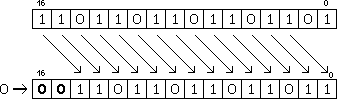version 2011-09-20

## SHR : logic SHift Right (divide unsigned by a power of two)

The bits of the source register (snd) are shifted towards the LSB, by a number of bits indicated either by

The MSB of the result are filled with 0s for this logic shift, while the arithmetic shift (SAR) fills the MSB with the value of the original MSB.

In the following examples, we will shift a register by two positions. This amounts to a division by 2^2=4. For YASEP16, the movement of the bits can be summarised by this diagram:For YASEP32, the principle is identical but with 32 bits instead of 16.

Let's consider that R1 gets loaded with 1101101101101101b, or 56173 in decimal. The unsigned shifting operation can be written this way:```mov 1101101101101101b r1
shr 2 r1```
56173/4=14043, which is equal to the binary result 11011011011011b.